SYLLABUS  Previous: 3 FORECASTING WITH UNCERTAINTY  Up: 3 FORECASTING WITH UNCERTAINTY  Next: 3.2 Simple valuation model

## 3.1 Option pricing for dummies

[ SLIDE forecasting - no arbitrage - uncertainty - eliminate risk || VIDEO login]

Because investors have different opinions about the value of every security that is traded in open markets, the spot price does not evolve smoothly with time and cannot be predicted with any certainty. Nevertheless, sect.2.1.1 showed that possible realizations can be simulated by adding price increments that are typical of volatilities from the past. This chapter examines increasingly sophisticated models to forecast market prices, using them first to estimate the average terminal payoff from financial derivatives, and later to calculate what is a fair price for an option before it expires.

For a qualitative understanding, you can think of an option as a form of insurance covering a financial risk without having any obligation: clearly, an insurance gets increasingly valuable when the market becomes risky and volatile... but how large should this risk premium be? To show here with a simple example how the price of options can be calculated, imagine a vanilla put expiring in 3 months (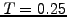years) with a strike price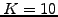, for an underlying currently valued at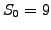EUR and risk free interest rate of 3% (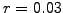).

In a first study, consider the case without drift nor volatility (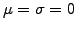) for which the forecast price simply remains constant at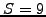EUR: the terminal payoff from the put option (2.1.3#eq.1) can then easily be calculated three months into the future as suggested by (3.1#fig.1) and yields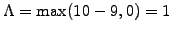EUR: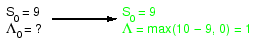A general no-arbitrage argument states that without taking any risk, a security has to grow exactly at the risk free interest (spot) rate: if the security grew more quickly, investors could make a risk less profit by borrowing money at the spot rate, buy large amounts of that security and sell it later for a higher price; on the contrary, if the security grew more slowly, investors could make a risk less profit by selling short large amounts of that security and re-invest the proceeds for the higher yield of the spot rate. This shows that a risk less investment always grow exactly at the risk free interest rate. In absence of volatility, the terminal payoff from the option can therefore be discounted back in time using the risk free rate during the entire lifetime of the option, which yields the present value of the option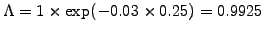.

In a second study, repeat the calculation using what appears to be a simplistic model of the uncertainty, where the forecast price can take only two distinct values.44Starting from the initial priceEUR, the sketch in (3.1#fig.2) shows how, after three months, the price of underlying can either move up to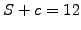or down to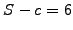EUR. The corresponding terminal option payoff are easily calculated and yield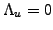and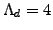.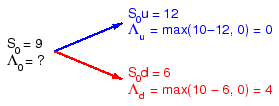Dealing with an uncertain outcome, the general strategy is to eliminate the risk by setting-up a perfectly hedged portfolio that combines an (a priori unknown) amount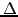of the underlying security with a (negatively correlated) derivative. The initial value of the portfolio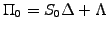evolves to new values until the expiry date, depending whether the underlying moves up or down. Choosing exactly the right hedging factor, it is however possible to force both outcomes to be equal, which in effect makes the investment risk less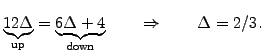(3.1#eq.1)

Indeed, the forecasted value of the portfolio in both cases becomes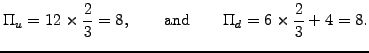(3.1#eq.2)

Repeating the general no-arbitrage argument, this a portfolio earns exactly the risk free interest rate and can be discounted back during the entire lifetime of the option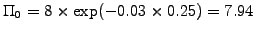. This has to be equal to the initial value of the portfolio: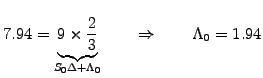(3.1#eq.3)

The example illustrates how the price of an option can be calculated before the expiry date, assuming a normal distribution of the price increments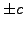that are typical for bonds. The study also shows that the price can be dramatically different when accounting for the uncertain evolution of the underlying. The next section takes these ideas further and shows how the price levels can be chosen to match the volatility observed in real markets.

SYLLABUS  Previous: 3 FORECASTING WITH UNCERTAINTY  Up: 3 FORECASTING WITH UNCERTAINTY  Next: 3.2 Simple valuation model